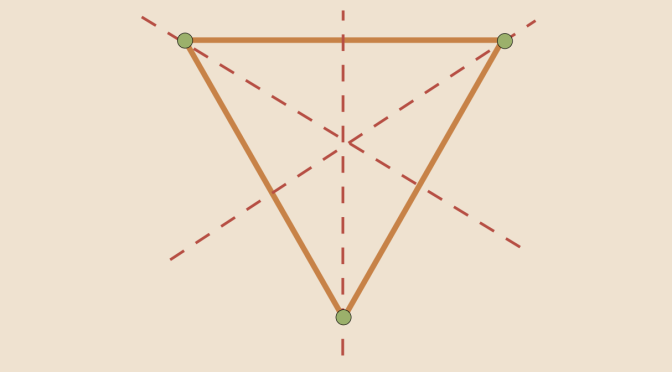Two subgroups whose product is not a subgroup

In this article, we consider a group $$G$$ and two subgroups $$H$$ and $$K$$. Let $$HK=\{hk \text{ | } h \in H, k \in K\}$$.

$$HK$$ is a subgroup of $$G$$ if and only if $$HK=KH$$. For the proof we first notice that if $$HK$$ is a subgroup of $$G$$ then it’s closed under inverses so $$HK = (HK)^{-1} = K^{-1}H^{-1} = KH$$. Conversely if $$HK = KH$$ then take $$hk$$, $$h^\prime k^\prime \in HK$$. Then $$(hk)(h^\prime k^\prime)^{-1} = hk(k^\prime)^{-1}(h^\prime)^{-1}$$. Since $$HK = KH$$ we can rewrite $$k(k^\prime)^{-1}(h^\prime)^{-1}$$ as $$h^{\prime \prime}k^{\prime \prime}$$ for some new $$h^{\prime \prime} \in H$$, $$k^{\prime \prime} \in K$$. So $$(hk)(h^\prime k^\prime)^{-1}=hh^{\prime \prime}k^{\prime \prime}$$ which is in $$HK$$. This verifies that $$HK$$ is a subgroup.

Also, if either $$H$$ or $$K$$ is a normal subgroup of $$G$$, $$HK$$ is a subgroup of $$G$$. This is true as if $$H$$ is normal in $$G$$ then we get that $$Hk = kk^{-1}Hk = kH$$ for every $$k \in K$$. Hence $$HK = KH$$ so it is a subgroup according to point above.

We now move to a counterexample of two subgroups whose product is not a subgroup. For $$G$$ we take the dihedral group $$D_3$$, the group of symmetries of an equilateral triangle. $$D_3 = \{i, r_1,r_2,s_1,s_2,s_3\}$$, here $$i$$ is the identity, $$r_1,r_2$$ the rotations at $$120$$ and $$240$$ degrees about the centroid, $$s_1, s_2, s_3$$ the axial symmetries with respect to medians. $$H=\{i,s_1\}$$ and $$K=\{i,s_2\}$$ are two subgroups of $$G$$ both of order $$2$$. Since the product of reflections (with intersecting axes) is a rotation, $$HK$$ will contain $$i$$, $$s_1$$, $$s_2$$ and a rotation. Therefore $$HK$$ has 4 distinct elements. $$HK$$ cannot be a subgroup as according to Lagrange’s theorem the order of a subgroup divides the order of the group.